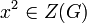# Center not is intermediately local powering-invariant in solvable group

This article gives the statement, and possibly proof, of the fact that in a group satisfying the property solvable group, the subgroup obtained by applying a given subgroup-defining function (i.e., center) need not satisfy a particular subgroup property (i.e., intermediately local powering-invariant subgroup)
View all such subgroup property satisfactions OR View more information on subgroup-defining functions in solvable groups

## Statement

It is possible to have a solvable group$G$ such that the center$Z(G)$ is not an intermediately local powering-invariant subgroup of$G$, i.e., there exists an intermediate subgroup$H$ of$G$ such that$Z(G)$ is not a local powering-invariant subgroup of$H$.

## Proof

Further information: amalgamated free product of Z and Z over 2Z

Consider the group$G = \mathbb{Z} *_{2\mathbb{Z}} \mathbb{Z}$, explicitly given as:$G := \langle x,y \mid x^2 = y^2 \rangle$

with the subgroup:$H = \langle x \rangle$

• The center of$G$ is the subgroup$Z(G) = \langle x^2 \rangle$, which is the amalgamated copy of$2\mathbb{Z}$.
•$G$ is solvable: In fact,$G/Z(G)$ is isomorphic to the infinite dihedral group, which is a metacyclic group.
• The subgroup$H = \langle x \rangle$ is isomorphic to$\mathbb{Z}$, with$Z(G)$ living as$2\mathbb{Z}$ inside it.
•$Z(G)$ is not local powering-invariant in$H$: To see this, note that the element$x^2 \in Z(G)$ has unique square root$x \in H$ but this square root is not in$Z(G)$.